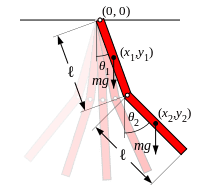# Lagrangian for released double pendulum

I just recently worked through the lagrangian method for describing the motion of a double pendulum. What I want to do now is describe the motion of a double pendulum that has been instantaneously released from the origin and allowed to fly through the air (with the 2 pendulums still connected to each other). I was hoping to get some direction on how to set-up this problem. A couple questions come to mind when I think about how to get started:

Where would I set the origin? In the case of the original double pendulum, the origin was set at axis of rotation of the first pendulum. Should I keep using that as my origin? Or maybe use the double pendulum's center of mass?

For the original double pendulum problem, only the linear kinetic energy is considered. In the case of a free flying double pendulum, would you consider rotational kinetic energy as well? Would I be considering the rotational energy about the individual pendulums center of mass or the rotational energy of the pendulums about the systems center of mass?

If my understanding of how to approach this problem is so far off the mark that answering these questions doesn't actually help find the solution, please let me know. And hopefully provide a little insight on where to begin.

Thanks,
TipTop

BiGyElLoWhAt
Gold Member
Wouldn't it be pretty hard to release a pendulum from where it's going to rotate about, and expect it to rotate about there?

In my experience with Lagrangians, you don't neglect the rotational KE, you can choose to express it in terms of linear translation, including a factor of r (which turns it into rotational KE) using v/r = omega. Also, I've found that that is the way to go. You get wierd "cross terms" that are hybrids of linearality and rotation when you start with cartesian and convert to polar or something. You likely won't be able to deduce those terms properly. (I wouldn't be able to anyway).

If I were you, I wouldn't release it from the origin. I would start it at some arbitrary point that is a distance of L (the length of the pendulum) away from the origin, then you can keep the origin at the axis of rotation.

Hey BiGyElLoWhAt,

Thanks for the reply. I just started setting up the lagrangian for a double pendulum with the origin at a distance L from the axis of rotation of the first pendulum. The equations of motion I get from this set up are that of 2 independent pendulums swinging about the point I chose as my origin. This, of course, is not the set of equations I desire. I think because the origin I've chosen is moving and accelerating (since it's attached to the end of the first pendulum) is a problem with this approach. Did I interpret your suggestion correctly?

I was thinking of putting the origin at some arbitrary point not on the pendulum and then describing the pendulums using their angles and their position relative to the center of mass of the system. My understanding is that upon releasing the double pendulum, the center of mass will travel in a parabolic shape, with the x-direction velocity being constant and the y-direction velocity accelerating by g. The math looks like it's going to be a little messy so I wanted to see if this approach made sense or not before doing all the calculations. Also, in this case, I'm not sure whether or not to take into account rotational kinetic energy.

As an aside, this problem involves pendulums which have their center of mass at the middle of their length.

Thanks,
TipTop.

BiGyElLoWhAt
Gold Member
I'm not sure that you did interpret my suggestion correctly. To make sure that I'm interpreting what you're trying to do correctly, let me ask: is a double pendulum a pendulum attached to the bob of another pendulum, both of which are free to rotate about where they are attached from? Also, as far as the rods go, massive, or massless; finally, bobs, dimensional or dimensionless?

Here's a picture of the double pendulum I'm imagining. Massive rods attached to each other by a pivot:This was taken from (http://en.wikipedia.org/wiki/Double_pendulum) where they describe how the set up the lagrangian for a double pendulum where the first pendulum is attached with a pivot to (0,0) and the second pendulum is attached with a pivot to the end of the first.

In the case shown in the picture above, the derivation for the lagrangian is described on wikipedia. What I want to know is how to set up the lagrangian for the case where the pivot attached to first pendulum and (0,0) disappears, allowing the double pendulum to fly away.

BiGyElLoWhAt
Gold Member
Ahh, you will have 2 lagrangians for the system. One for the time interval from when it is released to when the pivot breaks or "disappears", and another for when it is flying through the air. You need 2 because the system has different constraints over different intervals. Also, judging from the picture, I would say the rods are to be treated as 1 dimensional. So in the first situation, you'll have rotational KE, in the second you'll have translational and rotational.
Does that help? Also
I was thinking of putting the origin at some arbitrary point not on the pendulum and then describing the pendulums using their angles and their position relative to the center of mass of the system.
This seems like a reasonable start. The only thing I would say is that the equations of motion will be simpler if you place the origin at the point where the first pendulum is attached to the ceiling.

I think I've got enough now to give it a try. Thanks for the help!

BiGyElLoWhAt
Gold Member
No problem.